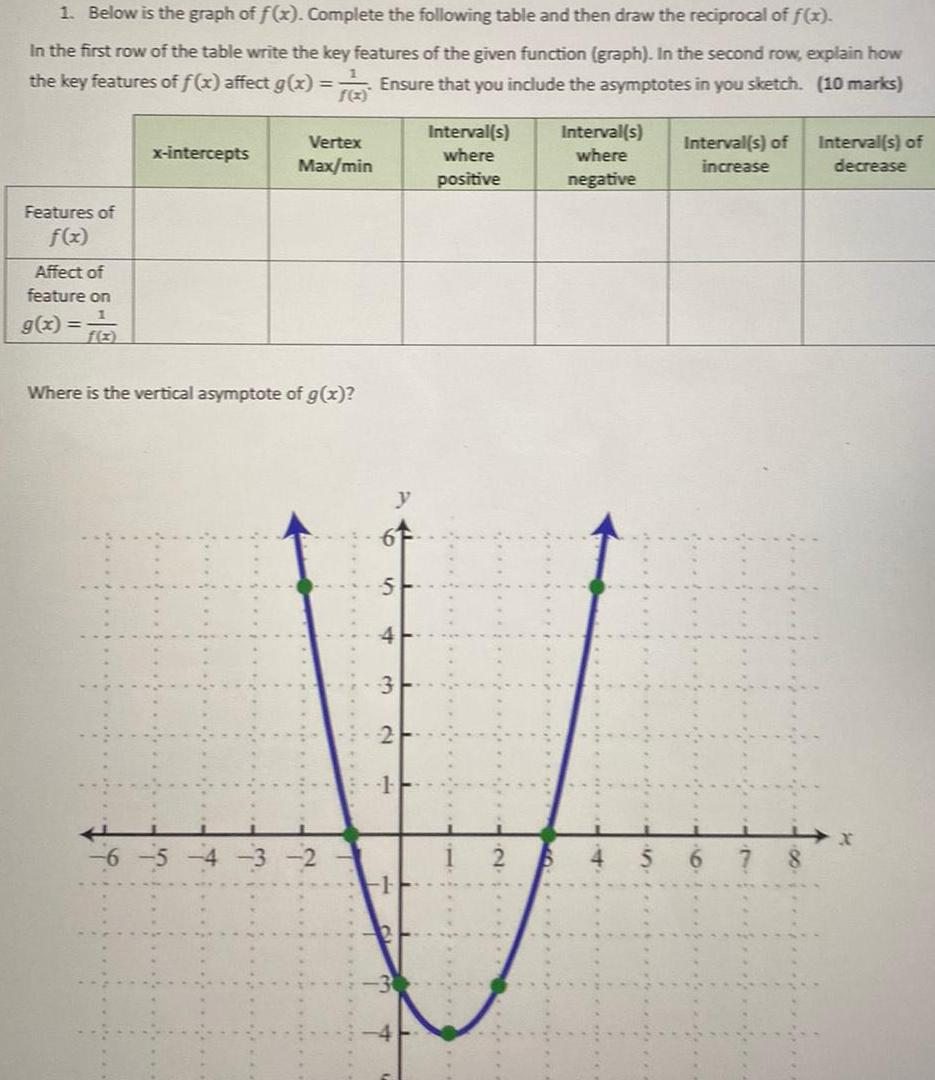Question:

# 1 Below is the graph of f x Complete the following table and

Last updated: 11/13/20231 Below is the graph of f x Complete the following table and then draw the reciprocal of f x In the first row of the table write the key features of the given function graph In the second row explain how the key features of f x affect g x Ensure that you include the asymptotes in you sketch 10 marks Features of f x Affect of feature on 1 g x x intercepts Vertex Max min Where is the vertical asymptote of g x S d 1 Interval s where positive Interval s where negative Interval s of increase Interval s of decrease